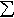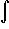#Interactive Real Analysis

Next | Previous | Glossary | Map

## 7.4. Lebesgue Integral

### Example 7.4.4(b): Lebesgue Integral for Simple Functions

Find the Lebesgue integral of a step function, i.e. a function s such that s(x) = cj for xj-1 < x < xj and the { xj } form a partition of [a, b].
A step function is a simple function, i.e.
s(x) =cj Xj(x)
where Xj is the characteristic function of the set (xj-1, xj). All sets are measurable with finite measure so that by definition:s(x) dx =cj m(Xj) =cj (xj - xj-1)
which is again the same answer as for the Riemann integral.
Next | Previous | Glossary | Map